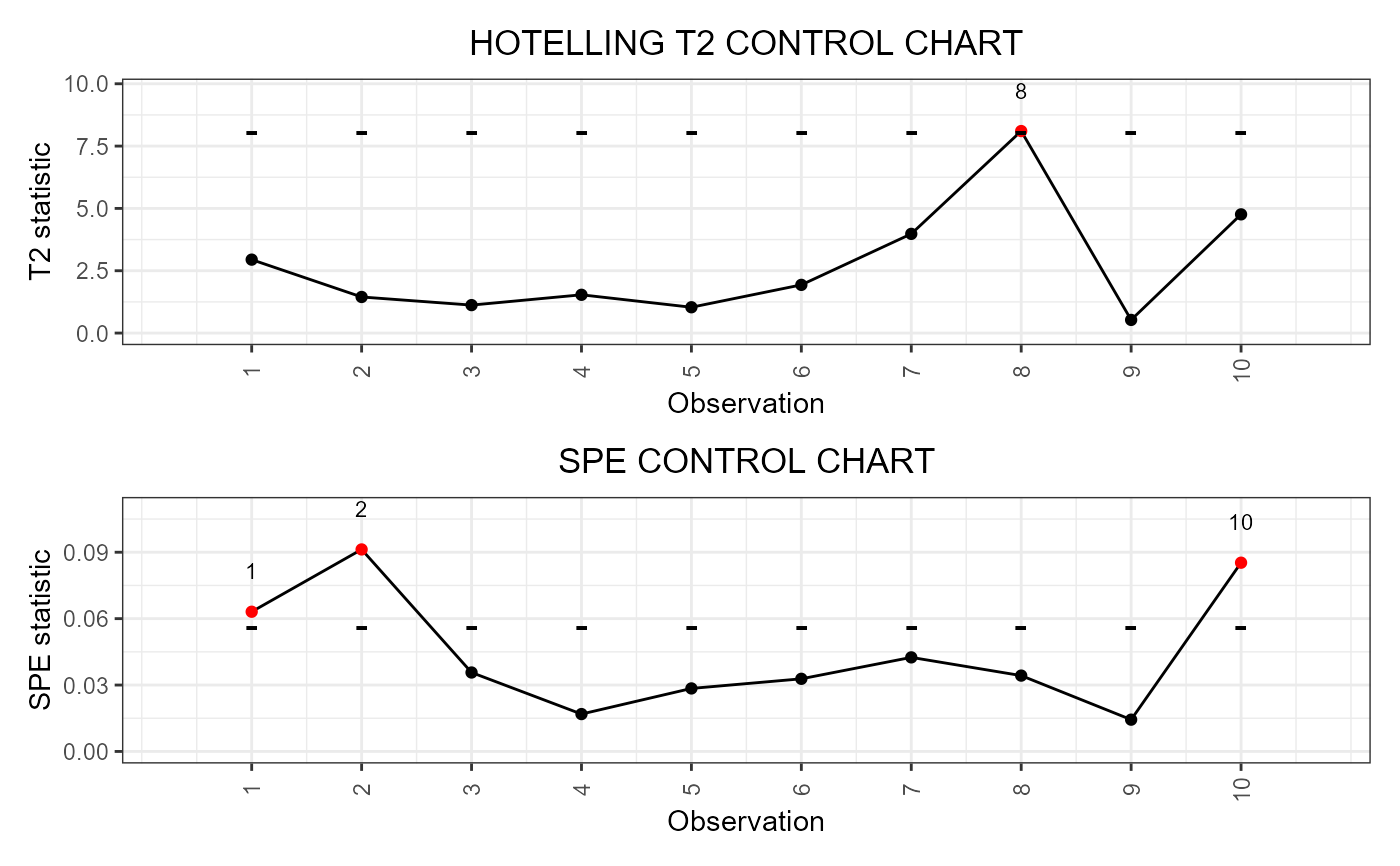It builds a data frame needed to plot the Functional Regression Control Chart introduced in Centofanti et al. (2021), for monitoring a functional quality characteristic adjusted for by the effect of multivariate functional covariates, based on a fitted function-on-function linear regression model. The training data have already been used to fit the model. An optional tuning data set can be provided that is used to estimate the control chart limits. A phase II data set contains the observations to be monitored with the control charts. It also allows to jointly monitor the multivariate functional covariates.

regr_cc_fof(
object,
mfdobj_y_new,
mfdobj_x_new,
mfdobj_y_tuning = NULL,
mfdobj_x_tuning = NULL,
alpha = 0.05,
include_covariates = FALSE,
absolute_error = FALSE
)

## Arguments

object

A list obtained as output from fof_pc, i.e. a fitted function-on-function linear regression model.

mfdobj_y_new

An object of class mfd containing the phase II data set of the functional response observations to be monitored.

mfdobj_x_new

An object of class mfd containing the phase II data set of the functional covariates observations to be monitored.

mfdobj_y_tuning

An object of class mfd containing the tuning data set of the functional response observations, used to estimate the control chart limits. If NULL, the training data, i.e. the data used to fit the function-on-function linear regression model, are also used as the tuning data set, i.e. mfdobj_y_tuning=object$pca_y$data. Default is NULL.

mfdobj_x_tuning

An object of class mfd containing the tuning data set of the functional covariates observations, used to estimate the control chart limits. If NULL, the training data, i.e. the data used to fit the function-on-function linear regression model, are also used as the tuning data set, i.e. mfdobj_x_tuning=object$pca_x$data. Default is NULL.

alpha

If it is a number between 0 and 1, it defines the overall type-I error probability. By default, it is equal to 0.05 and the Bonferroni correction is applied by setting the type-I error probabilities equal to alpha/2 in the Hotelling's T2 and SPE control charts. If include_covariates is TRUE, i.e., the Hotelling's T2 and SPE control charts are built also on the multivariate functional covariates, then the Bonferroni correction is applied by setting the type-I error probability in the four control charts equal to alpha/4. If you want to set manually the Type-I error probabilities, then the argument alpha must be a named list with elements named as T2, spe, T2_x and, spe_x, respectively, containing the desired Type I error probability of the T2 and SPE control charts for the functional response and the multivariate functional covariates, respectively.

include_covariates

If TRUE, also functional covariates are monitored through control_charts_pca,. If FALSE, only the functional response, conditionally on the covariates, is monitored.

absolute_error

A logical value that, if include_covariates is TRUE, is passed to control_charts_pca.

## Value

A data.frame containing the output of the function control_charts_pca applied to the prediction errors.

control_charts_pca

## Examples

library(funcharts)
data("air")
air <- lapply(air, function(x) x[1:100, , drop = FALSE])
fun_covariates <- c("CO", "temperature")
mfdobj_x <- get_mfd_list(air[fun_covariates],
n_basis = 15,
lambda = 1e-2)
mfdobj_y <- get_mfd_list(air["NO2"],
n_basis = 15,
lambda = 1e-2)
mfdobj_y1 <- mfdobj_y[1:60]
mfdobj_y_tuning <- mfdobj_y[61:90]
mfdobj_y2 <- mfdobj_y[91:100]
mfdobj_x1 <- mfdobj_x[1:60]
mfdobj_x_tuning <- mfdobj_x[61:90]
mfdobj_x2 <- mfdobj_x[91:100]
mod_fof <- fof_pc(mfdobj_y1, mfdobj_x1)
cclist <- regr_cc_fof(mod_fof,
mfdobj_y_new = mfdobj_y2,
mfdobj_x_new = mfdobj_x2,
mfdobj_y_tuning = NULL,
mfdobj_x_tuning = NULL)
plot_control_charts(cclist)